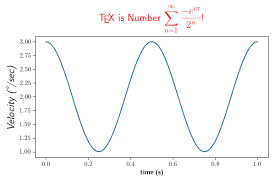## How to Calculate and Solve for TEX | Polymer & TextileThe image above represents TEX.

To compute for TEX, two essential parameters are needed and these parameters are mass (M) and length (L).

The formula for calculating TEX:

TEX = M/L x 1000

Where:

TEX = TEX
M = Mass
L = Length

Let’s solve an example;
Find the TEX when the mass is 8 and the length is 14.

This implies that;

M = Mass = 8
L = Length = 14

TEX = M/L x 1000
TEX = 8/14 x 1000
TEX = 0.57 x 1000
TEX = 571.4

Therefore, the TEX is 571.4.

Calculating the Mass when the TEX and the Length is Given.

M = TEX x L / 1000

Where:

M = Mass
TEX = TEX
L = Length

Let’s solve an example;
Find the mass when the TEX is 40 and the length is 8.

This implies that;

TEX = TEX = 40
L = Length = 8

M = TEX x L / 1000
M = 40 x 8 / 1000
M = 320 / 1000
M = 0.32

Therefore, the mass is 0.32.